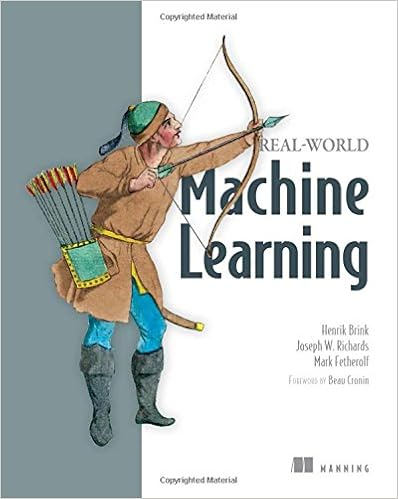# [Review] Real World Machine Learning

The book is divided into two parts. First part deals with machine learning workflow and the second part consists of five practical examples. The book is focused only on supervised machine learning, specifically regression, classification, recommendation and imputation. As the title says “real-world”, it contains examples in Python with scikit-learn and pandas libraries which are the most popular libraries for machine learning in Python.In the first chapter one can find what is machine learning and when it is useful. Next chapters cover data preprocessing, data visualization techniques and feature engineering. Reader can try classification of linear problem by logistic regression and non-linear problem by SVM. For regression problems linear regression and random forest is applied. Later chapters explain evaluation metrics, cross-validation and model optimization by brute-force searching of the hyper-parameters.

The second part of the book with practical examples is more interesting. Each chapter starts with an easy solution that is iteratively improved. First chapter covers whole machine learning pipeline, from exploring the data to solving the task of passenger tipping habits predictions on the NYC taxi data. Next two chapters are about advanced feature engineering with examples of extracting features from text (modelling reviews sentiment), images (modelling edges, shapes) and time-series data.

The algorithms are described at a high-level with no math at all. Moreover, there are best practices and references to dig deeper into each algorithm covered. Each chapter contains a vocabulary of terms and summary, which makes it clear what topics are covered in a chapter and it is possible to read each section separately.

To sum up, this book is a brief introduction to a machine learning. It gives you great overview of machine learning lifecycle with practical code examples for each part. I would recommend the book for all people proficient in Python who are interest to learn machine learning. When you read the book, you gain practical skills of preparing the data, extracting features and building a prediction model for several types of problems. It is a good starting point to begin exploring the machine learning world, because it covers most of the machine learning algorithms used in the industry. However, to know which algorithm to choose for a particular problem and how to choose the right hyper-parameters for a model, in my opinion you definitely need a theoretical knowledge of the algorithms. For me the first part of the book was easy and obvious, but second practical part was really great, especially the chapter about scalability.

Covered algorithms: logistic regression, linear regression, k-nearest neighbors, SVM, decision tree, random forest.

Tags:

Categories:

Updated: